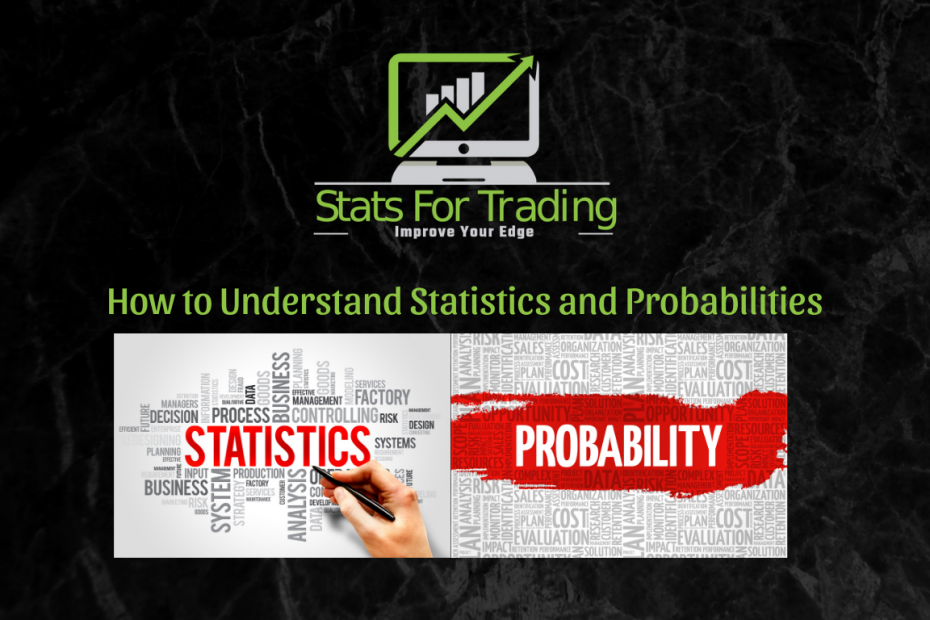# How to Understand Statistics and Probabilities## How to Understand Statistics and Probabilities

Let’s talk for a minute about understanding statistics and probabilities.  Let’s use the framework of the

Statistics

We have our range statistics. For instance, I take a period of time and I create statistics on the ranges of 30-minute periods.  I show you what the Median, Average, Max, and Min ranges are for a given number of days.  These are not probabilities.  They give a range of possibilities.  If we use the MAX range, for example, it may have only hit that range once in the given period of time, something more improbable than probable on any given day, but it is possible.  The range statistics help you to get to know a market, how it tends to behave on any given day or at any given time.

Probabilities

As part of our range statistics, I also publish the percentage of time that the current period will encompass, stay inside or close inside the prior period. Here is an example where a statistic becomes synonymous with a probability.  The statistic shows you the likelihood or probability of what will happen.

Here is something to remember about probabilities.  What is the root word of “probability”?  It is “probable”.  Let’s say you have a 100% probability of something happen.  Let’s say we have a statistic that says that Crude Oil, period 2 has a 100% probability of encompassing Period 1.  How could we state that in terms of understanding it in our decision-making process?  Simple.  You would say that Period 2 will “PROBABLY” encompass Period 1.  A 100% probability is not the same as a 100% guarantee.  Do not think in terms of what will happen but in terms of what is likely to happen or in terms of what will probably happen.

Just think of the emotional difference in understanding this.  If you think you have a 100% chance of something happening and it doesn’t, what emotions may you experience if your trade fails?  Anger, betrayal, mistrust!  Now think about it in terms of something that will probably happen.  If the trade fails, sure you are disappointed, but are you angry, do you lose faith?  Hopefully not.  Learning to think in probabilities correctly can help preserve your emotional energy and keep you trading.